# C语言 位运算符

C 语言支持的位运算符直接对bit位进行操作，其效率最高，总共有6种位运算符：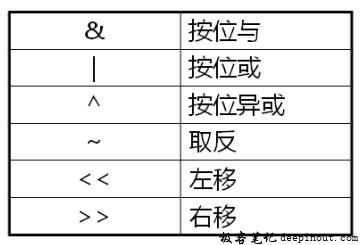& 按位与操作，按二进制位进行”与”运算。运算规则：0&0=0; 0&1=0; 1&0=0; 1&1=1; (A & B) 将得到 12，即为 0000 1100
| 按位或运算符，按二进制位进行”或”运算。运算规则：0|0=0; 0|1=1; 1|0=1; 1|1=1; (A | B) 将得到 61，即为 0011 1101
^ 异或运算符，按二进制位进行”异或”运算。运算规则：0^0=0; 0^1=1; 1^0=1; 1^1=0; (A ^ B) 将得到 49，即为 0011 0001
~ 取反运算符，按二进制位进行”取反”运算。运算规则：~1=0; ~0=1; (~A ) 将得到 -61，即为 1100 0011，一个有符号二进制数的补码形式。
<< 二进制左移运算符。将一个运算对象的各二进制位全部左移若干位（左边的二进制位丢弃，右边补0）。 A << 2 将得到 240，即为 1111 0000
>> 二进制右移运算符。将一个数的各二进制位全部右移若干位，正数左补0，负数左补1，右边丢弃。 A >> 2 将得到 15，即为 0000 1111

## 左移和右移

• 左操作数必须为整数类型：char和short被隐式转换为int后进行移位操作
• 右操作数的范围为:[0,31] (32bit操作系统)，如果右操作数小于0，则会产生随机的结果
• 左移运算符<<将运算数的二进制位左移，移动规则是：高位丢弃，低位补0
• 右移运算法>>将运算数的二进制位右移，移动规则是：高位补符号位，低位丢弃

### 示例：位运算符初探

#include <stdio.h>

int main()
{
printf("%d\n", 3 << 2);
printf("%d\n", 3 >> 1);
printf("%d\n", -1 >> 1); // -1所有位都是1, 右移还都是1
printf("%d\n", 0x01 << 2 + 3); // 位运算符 优先级低于 四则运算符

printf("%d\n", 3 << -1); // oops!，具体的结果由编译器决定，为不确定值

return 0;
}


gcc运行结果：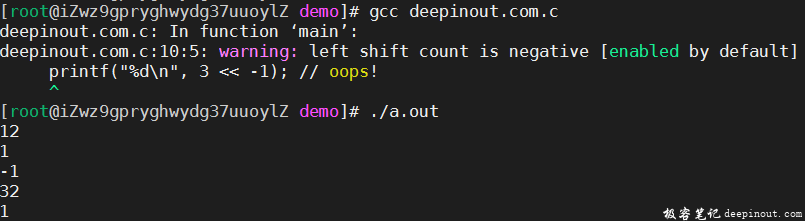vc10.0运行结果，可知3 << -1的结果为0：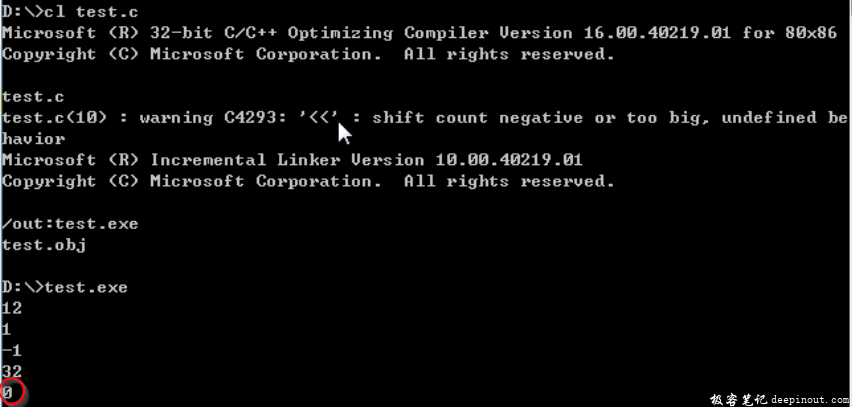## 位运算法使用技巧

• 避免位运算符、逻辑运算符和数学运算符同时出现在一个表达式中
• 当位运算符、逻辑运算符和数学运算符需要同时参与运算时，尽量使用括号()来表达计算次序
• 左移n位相当于乘以2的n次方，效率比数学运算符高
• 右移n位相当于除以2的n次方，效率比数学运算符高

## 示例：交换两个整型变量的值

SWAP1显示不满足要求了。

SWAP2虽然没有借助中间变量，但当a和b都是非常大的整数时，那么相加后的结果可能会产生溢出，这时，这种方法还是有问题。

SWAP3才是最完美的写法！

#include <stdio.h>

#define SWAP1(a, b)    \
{                      \
int t = a;         \
a = b;             \
b = t;             \
}

#define SWAP2(a, b)    \
{                      \
a = a + b;         \
b = a - b;         \
a = a - b;         \
}

#define SWAP3(a, b)    \
{                      \
a = a ^ b;         \
b = a ^ b;         \
a = a ^ b;         \
}

int main()
{
int a = 1;
int b = 2;

printf("a = %d\n", a);
printf("b = %d\n", b);

SWAP3(a ,b);

printf("a = %d\n", a);
printf("b = %d\n", b);

return 0;
}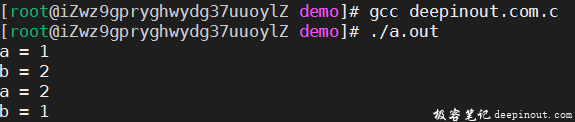## 位运算符与逻辑运算符

• 位运算符没有短路规则，每个操作数都参与运算
• 位运算符的结果为整数，而不是0或1
• 位运算符优先级高于逻辑运算符

## 示例：混淆概念的判断条件

#include <stdio.h>

int main()
{
int i = 0;
int j = 0;
int k = 0;

if( ++i | ++j & ++k )
{
printf("Run here...\n");
}

return 0;
}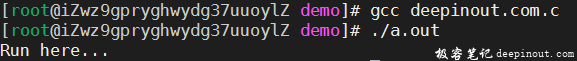i，j，k的值也都是1了，也就是说为运算符没有短路规则，注意不要与||,&&混淆了概念。

## 总结

• 位运算符只能用于整数类型
• 左移和右移运算符的右操作数范围必须为[0,31]（32bit操作系统）
• 位运算符没有短路规则，所有操作数均会求值
• 位运算符的效率高于四则运算符和逻辑运算符
• 运算符优先级：四则运算>位运算>逻辑运算

• 微信订阅
• 回顶